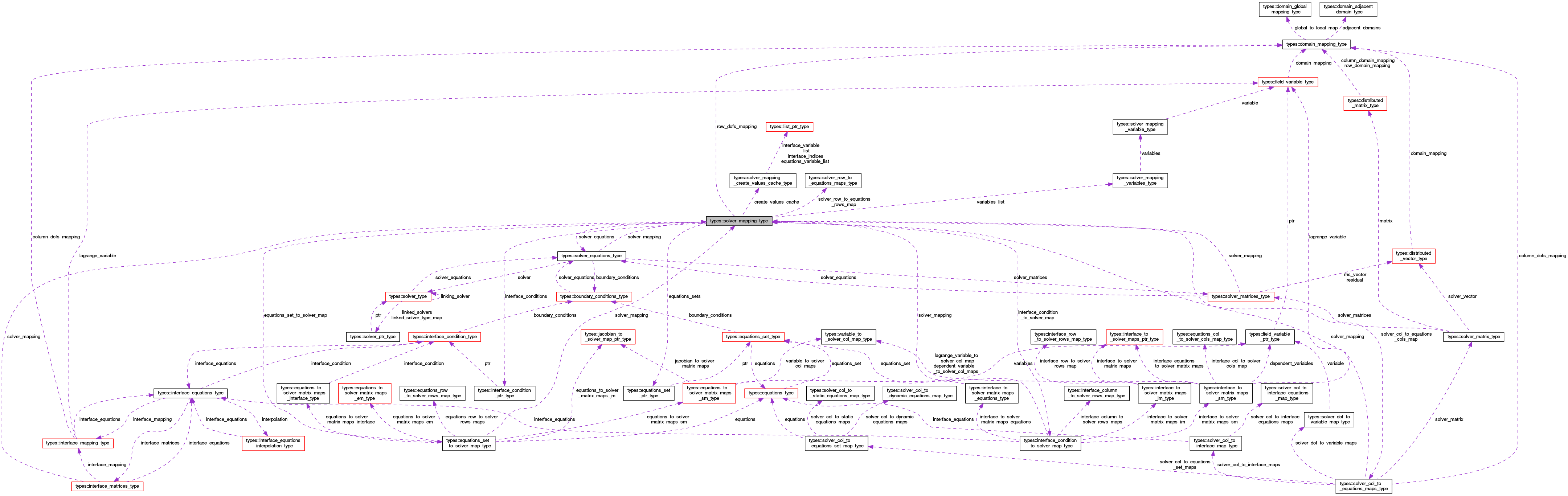OpenCMISS-Iron Internal API Documentation
types::solver_mapping_type Type Reference

Contains information on the solver mapping between the global equation sets and the solver matrices. More...

Collaboration diagram for types::solver_mapping_type:[legend]

## Public Attributes

type(solver_equations_type), pointer solver_equations
A pointer to the solver equations for this mapping. More...

logical solver_mapping_finished
Is .TRUE. if the solution mapping has finished being created, .FALSE. if not. More...

integer(intg) number_of_solver_matrices
The number of solution matrices in this mapping. More...

integer(intg) number_of_rows
The number of (local) rows in the solver matrices. More...

integer(intg) number_of_global_rows
The number of global rows in the solver matrices. More...

integer(intg) number_of_equations_sets
The number of equations sets in the solution mapping. More...

type(equations_set_ptr_type), dimension(:), allocatable equations_sets
The list of equations sets that are in this solution mapping. More...

type(equations_set_to_solver_map_type), dimension(:), allocatable equations_set_to_solver_map
EQUATIONS_SET_TO_SOLVER_MAP(equations_set_idx). The mapping from the equations_set_idx'th equations set to the solver matrices. More...

integer(intg) number_of_interface_conditions
The number of interface conditions in the solution mapping. More...

type(interface_condition_ptr_type), dimension(:), allocatable interface_conditions
The list of interface conditions that are in this. More...

type(interface_condition_to_solver_map_type), dimension(:), allocatable interface_condition_to_solver_map
INTERFACE_CONDITION_TO_SOLVER_MAP(interface_condition_idx). The mapping from the interface_condition_idx'th interface condition to the solver matrices. More...

type(solver_mapping_variables_type), dimension(:), allocatable variables_list
VARIABLES_LIST(solver_matrix_idx). The list of variable for the solver_matrix_idx'th solver matrix. More...

type(solver_col_to_equations_maps_type), dimension(:), allocatable solver_col_to_equations_cols_map
SOLVER_TO_EQUATIONS_SET_MAP(solver_matrix_idx). The mapping from the solver_matrix_idx'th solver matrix to the equations set. More...

type(solver_row_to_equations_maps_type), dimension(:), allocatable solver_row_to_equations_rows_map
SOLVER_ROW_TO_EQUATIONS_SET_MAPS(local_row_idx). The mappings from the local_row_idx'th solver row to the equations set rows. More...

type(domain_mapping_type), pointer row_dofs_mapping
The domain mapping for the solver rows. More...

type(solver_mapping_create_values_cache_type), pointer create_values_cache
The create values cache for the solver mapping. More...

## Detailed Description

Contains information on the solver mapping between the global equation sets and the solver matrices.

Definition at line 3091 of file types.f90.

## Member Data Documentation

 type(solver_mapping_create_values_cache_type), pointer types::solver_mapping_type::create_values_cache

The create values cache for the solver mapping.

Definition at line 3108 of file types.f90.

 type(equations_set_to_solver_map_type), dimension(:), allocatable types::solver_mapping_type::equations_set_to_solver_map

EQUATIONS_SET_TO_SOLVER_MAP(equations_set_idx). The mapping from the equations_set_idx'th equations set to the solver matrices.

Definition at line 3099 of file types.f90.

 type(equations_set_ptr_type), dimension(:), allocatable types::solver_mapping_type::equations_sets

The list of equations sets that are in this solution mapping.

Definition at line 3098 of file types.f90.

 type(interface_condition_to_solver_map_type), dimension(:), allocatable types::solver_mapping_type::interface_condition_to_solver_map

INTERFACE_CONDITION_TO_SOLVER_MAP(interface_condition_idx). The mapping from the interface_condition_idx'th interface condition to the solver matrices.

Definition at line 3102 of file types.f90.

 type(interface_condition_ptr_type), dimension(:), allocatable types::solver_mapping_type::interface_conditions

The list of interface conditions that are in this.

Definition at line 3101 of file types.f90.

 integer(intg) types::solver_mapping_type::number_of_equations_sets

The number of equations sets in the solution mapping.

Definition at line 3097 of file types.f90.

 integer(intg) types::solver_mapping_type::number_of_global_rows

The number of global rows in the solver matrices.

Definition at line 3096 of file types.f90.

 integer(intg) types::solver_mapping_type::number_of_interface_conditions

The number of interface conditions in the solution mapping.

Definition at line 3100 of file types.f90.

 integer(intg) types::solver_mapping_type::number_of_rows

The number of (local) rows in the solver matrices.

Definition at line 3095 of file types.f90.

 integer(intg) types::solver_mapping_type::number_of_solver_matrices

The number of solution matrices in this mapping.

Definition at line 3094 of file types.f90.

 type(domain_mapping_type), pointer types::solver_mapping_type::row_dofs_mapping

The domain mapping for the solver rows.

Definition at line 3107 of file types.f90.

 type(solver_col_to_equations_maps_type), dimension(:), allocatable types::solver_mapping_type::solver_col_to_equations_cols_map

SOLVER_TO_EQUATIONS_SET_MAP(solver_matrix_idx). The mapping from the solver_matrix_idx'th solver matrix to the equations set.

Definition at line 3104 of file types.f90.

 type(solver_equations_type), pointer types::solver_mapping_type::solver_equations

A pointer to the solver equations for this mapping.

Definition at line 3092 of file types.f90.

 logical types::solver_mapping_type::solver_mapping_finished

Is .TRUE. if the solution mapping has finished being created, .FALSE. if not.

Definition at line 3093 of file types.f90.

 type(solver_row_to_equations_maps_type), dimension(:), allocatable types::solver_mapping_type::solver_row_to_equations_rows_map

SOLVER_ROW_TO_EQUATIONS_SET_MAPS(local_row_idx). The mappings from the local_row_idx'th solver row to the equations set rows.

Definition at line 3105 of file types.f90.

 type(solver_mapping_variables_type), dimension(:), allocatable types::solver_mapping_type::variables_list

VARIABLES_LIST(solver_matrix_idx). The list of variable for the solver_matrix_idx'th solver matrix.

Definition at line 3103 of file types.f90.